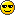# Vanish Clan Members##Vanish Clan Members

Here is the list of the current members in Vanish Clan

1) ==[Vanish] My Wineglass Hero ==
2) ==[Vanish] Minister ==
3) ==[Vanish] Rocket Prince ==
4) ==[Vanish] Stealthkiller(+) ==
5) ==[Vanish] Houlistic ==
6) ==[Vanish] Luffy|Zoro
7) ==[Vanish] mnb ====[Vanish] Wayne ==
9) ==[Vanish] #Hamster|Juice ==
10) ==[Vanish] Camillia ==
11) ==[Vanish] Iceman ==
12) ==[Vanish] C.Ronaldo ==
13) ==[Vanish] Retrisexion ==
14) ==[Vanish] Deathraider ==
15) ==[Vanish] Verizon ==
16) ==[Vanish] Ishagz ==
17) ==[Vanish] Jonny ==
18) ==[Vanish] Dragon|Heart ==
19) ==[Vanish] Noobear ==
20) ==[Vanish] Skill.ded ==
21) ==[Vanish] Timbuktoo ==
22) ==[Vanish] Vendetta ==
23) ==[Vanish] Ayumi/Neffy ==
24) ==[Vanish] Ch0c0 ==
25) ==[Vanish] Kartik ==
26) ==[Vanish] Darym ==
27) ==[Vanish] Oliver ==
28) ==[Vanish] excretions ==
29) ==[Vanish] Combomeal == (Reason: Quit cz (bought new toy to play wif))
30) ==[Vanish] Genesis ==
*As of 30th Oct 2008

Last edited by Rocket Prince on Sat Oct 25, 2008 11:26 pm; edited 1 time in totalPermissions in this forum:
You cannot reply to topics in this forum